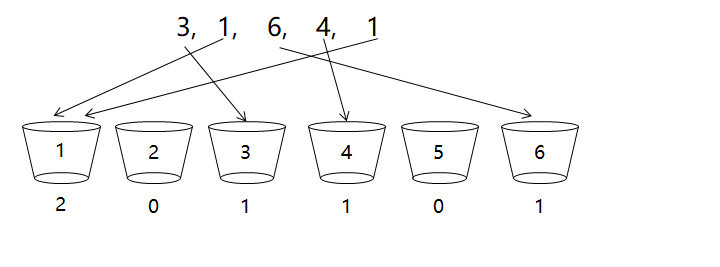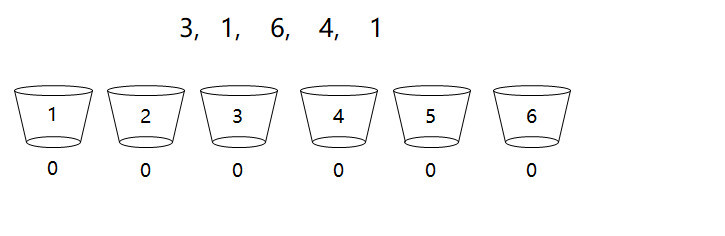# 手写计数排序算法1，1， 3， 4， 6

void counting_sort(int* parr, int count) {    // 1. find min and max value    int min = parr;    int max = parr;    for (int i = 1; i < count; i++) {        if (parr[i] < min) {            min = parr[i];        } else if (parr[i] > max) {            max = parr[i];        }    }    // 2. alloc space for counting    int n = max - min + 1;    int* pbuf = (int*)malloc(n * sizeof(int));    memset(pbuf, 0, n * sizeof(int));    // 3. counting    for (int i = 0; i < count; i++) {        pbuf[parr[i] - min]++;    }    // 4. generate sorting array    int idx = 0;    for (int i = 0; i < n; i++) {        if (pbuf[i] < 1) continue;​        for (int k = 0; k < pbuf[i]; k++) {            parr[idx++] = min + i;        }    }    // 5. free space    free(pbuf);}## 评论